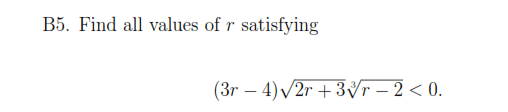# Solve Inequalities algebraically

Panphobia

## Homework Statement## The Attempt at a Solution

So when I started this question, I knew that 2r +3 > 0 in order for this whole thing to work so r > -3/2, and I know that if the middle term has to be more than 0, than only one more of the others is going to be negative, for the first term i found that r < 4/3 and the third was r < 2, so I know that the answer should be (4/3, 2), but how do I prove that algebraically?

Last edited:

Homework Helper
So when I started this question, I knew that 2r +3 > 0 in order for this whole thing to work so r > -3/2, and I know that if the middle term has to be more than 0, than only one more of the others is going to be negative, for the first term i found that r < 4/3 and the third was r < 2, so I know that the answer should be (4/3, 2), but how do I prove that algebraically?
I wouldn't solve it "algebraically." I would solve it by making a two-column table. First, find values of r that would make the left side 0 or undefined (I call these critical values), which appears you have already done. Then in the first column list all critical values and all intervals bounded by these critical values.
Code:
Test interval|Value
-------------+----------
(-inf, -3/2) |undefined
r = -3/2     |0
(-3/2, 4/3)  |
r = 4/3      |0
(4/3, 2)     |
r = 2        |0
(2, inf)     |
In the second column, you fill in 0, undefined, positive, or negative. For the remaining intervals, pick a test value to plug into the inequality and you will eventually determine the solution set.

Panphobia
ohhhh ok, that makes sense. thank you.

Homework Helper
Since the square root, $\sqrt{2r+ 3}$, is, by definition, nonnegative, you can (almost) ignore it. The product of the two numbers, 3r- 4 and $\sqrt{r- 2}$, will be negative if and only if the two factors are of opposite order. In other words, either

1) 3r- 4> 0 and $\sqrt{r- 2}< 0$ or
2) 3r- 4< 0 and $\sqrt{r- 2}> 0$

Similarly, a positive number, cubed, is positive and a negative number, cubed, is negative. So that can be reduced to
1) 3r- 4> 0 and r- 2< 0 or
2) 3r- 4< 0 and r- 2> 0.

3r- 4> 0 for r> 4/3 and r- 2< 0 for r< 3. Those will both be true for 4/3< r< 3.
$\sqrt{2r+ 3}= 0$ only for r= -3/2 and that does not lie between 4/3 and 3.

(1) is true for 4/3< r< 3.

3r- r< 0 for r< 4/3 and r- 2> 0 for r> 3. (2) is never true.